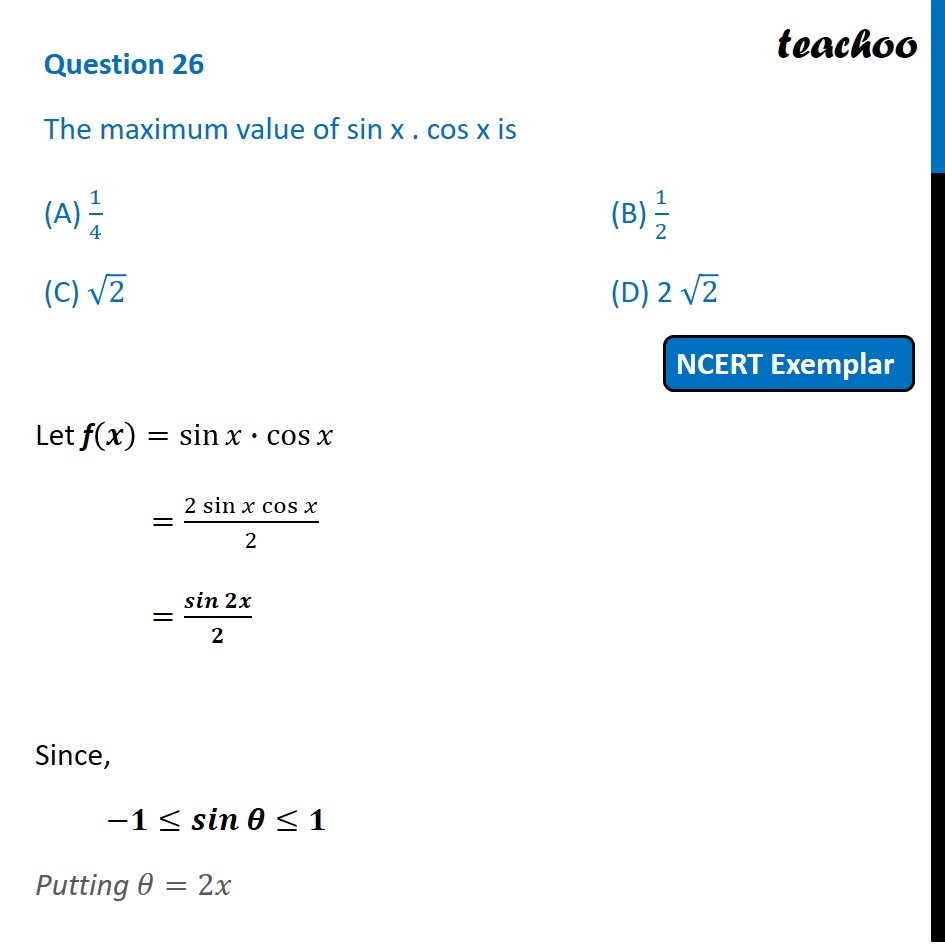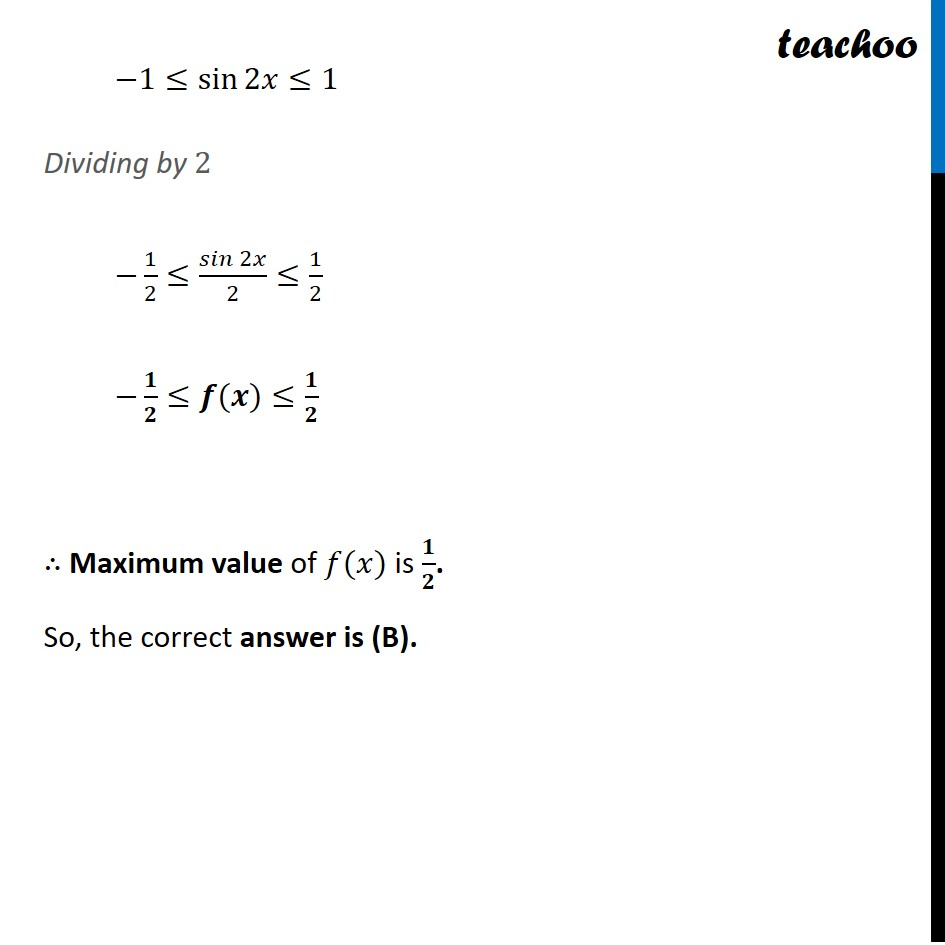NCERT Exemplar - MCQs

Chapter 6 Class 12 Application of Derivatives
Serial order wise

## (C) √2                      (D) 2 √2

This question is similar to Ex 6.5, 9 - Chapter 6 Class 12 - Application of DerivativesLearn in your speed, with individual attention - Teachoo Maths 1-on-1 Class

### Transcript

Question 13 The maximum value of sin x . cos x is (A) 1/4 (B) 1/2 (C) √2 (D) 2 √2 Let f(𝒙)=sin⁡𝑥∙cos⁡𝑥 =(2 sin⁡𝑥 cos⁡𝑥)/2 =(𝒔𝒊𝒏 𝟐𝒙)/𝟐 Since, −𝟏≤𝒔𝒊𝒏 𝜽≤𝟏 Putting 𝜃=2𝑥 −1≤sin⁡2𝑥≤1 Dividing by 2 −1/2≤(𝑠𝑖𝑛 2𝑥)/2≤1/2 −𝟏/𝟐≤𝒇(𝒙)≤𝟏/𝟐 ∴ Maximum value of 𝑓(𝑥) is 𝟏/𝟐. So, the correct answer is (B).